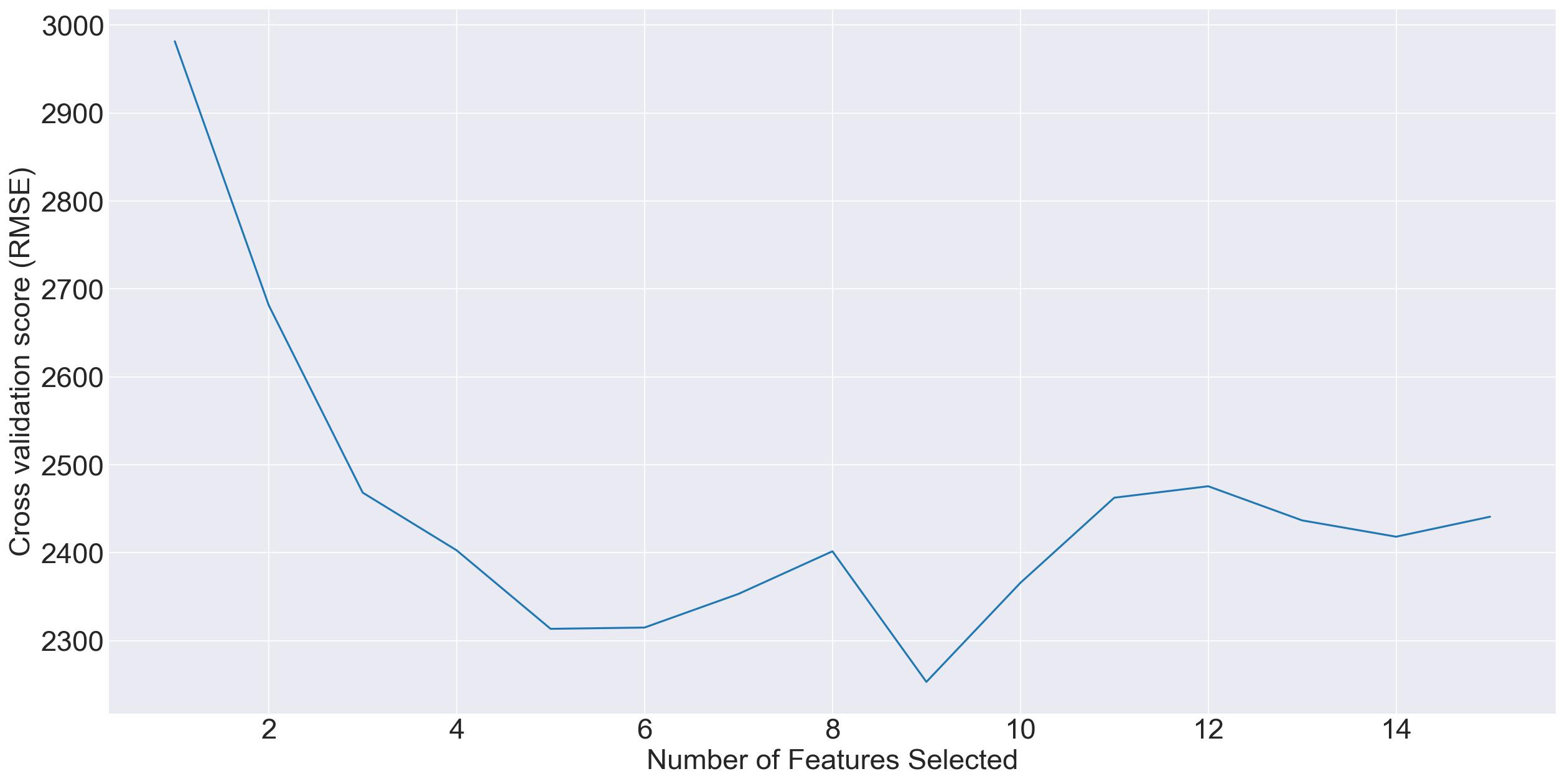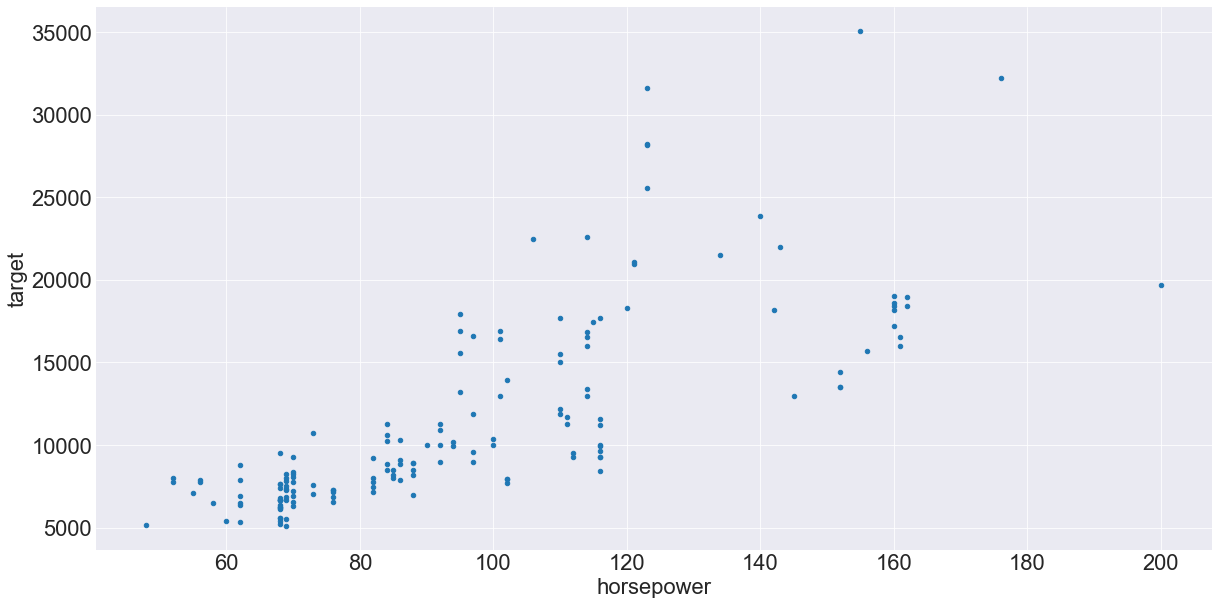# Feature Selection with a Scikit-Learn Pipeline

## March 25, 2018

I am a big fan of scikit-learn’s pipelines. Why are pipelines useful?

• Ensure reproducibility
• Export models to JSON for production models
• Enforce structure in preprocessing and hyperparameter search to avoid over-optimistic error estimates

Unfortunately though, there are a number of sklearn modules not well integrated with pipelines. In particular, feature selection. No doubt you’ve encountered:

RuntimeError: The classifier does not expose "coef_" or "feature_importances_" attributes


After a lot of digging, I managed to make feature selection work with a small extension to the Pipeline class.

Before we get started, some details about my setup:

• Python 3.6.4
• scikit-learn 0.19.1
• pandas 0.22.0

First, some boilerplate:

from sklearn import feature_selection
from sklearn import preprocessing
from sklearn.model_selection import StratifiedKFold
from sklearn.ensemble import ExtraTreesRegressor
from sklearn.pipeline import Pipeline
import numpy as np
import pandas as pd

from pmlb import fetch_data

import matplotlib.pyplot as plt
import seaborn as sns
%matplotlib inline

sns.set_style("darkgrid")


Let’s use the 195_auto_price regression data set from the Penn Machine Learning Benchmarks. The data set consists of prices for 159 vehicles as well as 15 numeric features about the vehicles. Details here.

X, y = fetch_data('195_auto_price', return_X_y=True)

feature_names = (
fetch_data('195_auto_price', return_X_y=False)
.drop(labels="target", axis=1)
.columns
)
feature_names

# Index(['symboling', 'normalized-losses', 'wheel-base', 'length', 'width',
#       'height', 'curb-weight', 'engine-size', 'bore', 'stroke',
#       'compression-ratio', 'horsepower', 'peak-rpm', 'city-mpg',
#       'highway-mpg'],
#      dtype='object')


Next, we’ll make a simple pipeline that:

• Standardizes features with zero mean and unit variance
• Training an extremely randomized tree regression model with 250 trees (default hyperparameters)
pipe = Pipeline(
[
('std_scaler', preprocessing.StandardScaler()),
("ET", ExtraTreesRegressor(random_state=42, n_estimators=250))
]
)


For this exercise, we’ll select features with recursive feature elimination. To select the features, we’ll choose the number of features that minimizes the mean squared error (MSE) based on 10-fold cross-validation.

feature_selector_cv = feature_selection.RFECV(pipe, cv=10, step=1, scoring="neg_mean_squared_error")
feature_selector_cv.fit(X, y)

# RuntimeError: The classifier does not expose "coef_" or "feature_importances_" attributes


BOOM! What happened? The Pipeline object itself does not contain the standard attributes coef_ or feature_importances_. So what do we do?

To address the problem, we extend the Pipeline class and create a new PipelineRFE. When the RFECV object is fit, the feature_importances_ attribute is extracted from the ExtraTreesRegressor and assigned to the PipelineRFE object.

class PipelineRFE(Pipeline):

def fit(self, X, y=None, **fit_params):
super(PipelineRFE, self).fit(X, y, **fit_params)
self.feature_importances_ = self.steps[-1][-1].feature_importances_
return self


So now, let’s rerun the same code as above, but this time we’ll create a PipelineRFE object.

pipe = PipelineRFE(
[
('std_scaler', preprocessing.StandardScaler()),
("ET", ExtraTreesRegressor(random_state=42, n_estimators=250))
]
)

# Sets RNG seed to reproduce results (your results should match mine)
_ = StratifiedKFold(random_state=42)

feature_selector_cv = feature_selection.RFECV(pipe, cv=10, step=1, scoring="neg_mean_squared_error")
feature_selector_cv.fit(X, y)

feature_selector_cv.n_features_
# 9


Fantastic! It worked, and 9 features were selected as the optimal number.

Now, let’s take a look at the cross-validated RMSE scores (If you’re unfamiliar with sklearn, the error is maximized, so the scores must be negated in order to be minimized). You should get the same values as I did because we set the random_state=42 above.

cv_grid_rmse = np.sqrt(-feature_selector_cv.grid_scores_)
cv_grid_rmse

# array([2981.48089461, 2681.34632497, 2468.32368972, 2402.74458326,
#        2313.48908854, 2314.93752784, 2353.19070845, 2401.63239588,
#        2253.1618682 , 2365.70835985, 2462.56697442, 2475.59320584,
#        2436.7582986 , 2418.22783946, 2440.94259292])


We can also plot the cross-validated RMSE scores to see that 9 features was indeed optimal.So which features were selected?

selected_features = feature_names[feature_selector_cv.support_].tolist()
selected_features

# ['wheel-base',
#  'length',
#  'width',
#  'curb-weight',
#  'engine-size',
#  'horsepower',
#  'peak-rpm',
#  'city-mpg',
#  'highway-mpg']


None of the features are surprising, and they all appear to be correlated with vehicle prices. For instance, here’s a scatterplot of horsepower and price:

fetch_data('195_auto_price', return_X_y=False).plot.scatter("horsepower", "target", figsize=(20, 10))And now, to make the pipeline copy/paste friendly, here’s the entire code block:

from sklearn import feature_selection
from sklearn import preprocessing
from sklearn.model_selection import StratifiedKFold
from sklearn.ensemble import ExtraTreesRegressor
from sklearn.pipeline import Pipeline
import numpy as np
import pandas as pd

from pmlb import fetch_data

import matplotlib.pyplot as plt
import seaborn as sns
%matplotlib inline

sns.set_style("darkgrid")

class PipelineRFE(Pipeline):

def fit(self, X, y=None, **fit_params):
super(PipelineRFE, self).fit(X, y, **fit_params)
self.feature_importances_ = self.steps[-1][-1].feature_importances_
return self

pipe = PipelineRFE(
[
('std_scaler', preprocessing.StandardScaler()),
("ET", ExtraTreesRegressor(random_state=42, n_estimators=250))
]
)

# Sets RNG seed to reproduce results (your results should match mine)
_ = StratifiedKFold(random_state=42)

feature_selector_cv = feature_selection.RFECV(pipe, cv=10, step=1, scoring="neg_mean_squared_error")
feature_selector_cv.fit(X, y)

comments powered by Disqus